## Example Questions

← Previous 1 3

### Example Question #1 : How To Convert Decimals To Scientific Notation

Write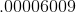in scientific notation.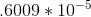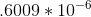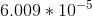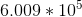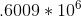Explanation:

We want to move the decimal point to the place just after the first non-zero number, in this case 6, and then drop all of the non-significant zeros. We need to move the decimal point five spaces to the right, so our exponent should be negative.  If the decimal had moved left, we would have had a positive exponent.

In this case we get 6.009 * 105.

### Example Question #1 : How To Convert Decimals To Scientific Notation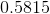is equal to which of the following?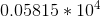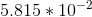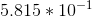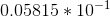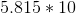Explanation:

We need to convertinto a number of the form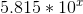.

The trick is, however, figuring out whatshould be. When you have to move your decimal point to the right, you need to make the decimal negative. (Note, though, when you multiply by a negative decimal, you move to the left. We are thinking in "reverse" because we are converting.)

Therefore, for our value,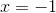. So, our value is:### Example Question #1 : How To Convert Decimals To Scientific Notation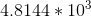is equal to which of the following?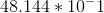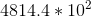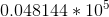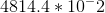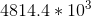Explanation:

The easiest way to do this is to convert each of your answer choices into scientific notion and compare it to.

For each of the answer choices, this would give us: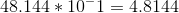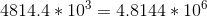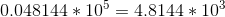(Which is, thus, the answer.)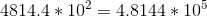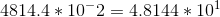When you convert, you add for each place that you move to the left and subtract for each place you move to the right. (Note that this is opposite of what you do when you multiply out the answer. We are thinking in "reverse" because we are converting.)

### Example Question #1 : How To Convert Decimals To Scientific Notation

Convert the decimal into scientific notation: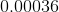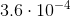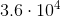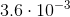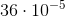Explanation:

To convert a decimal into scientific notation, move the decimal point until you get to the left of the first non-zero integer. The number of places the decimal point moves is the power of the exponent, because each movement represents a "power of 10". The exponent will be positive if the original number is greater than zero, and negative if the original number is less than zero.

For this example, move the decimal point four places to the right. Since the number is less than zero, the exponent is negative: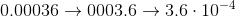### Example Question #1 : How To Convert Decimals To Scientific Notation

Convert the decimal into scientific notation: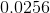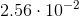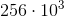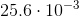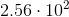Explanation:

To convert a decimal into scientific notation, move the decimal point until you get to the left of the first non-zero integer. The number of places the decimal point moves is the power of the exponent, because each movement represents a "power of 10". The exponent will be positive if the original number is greater than zero, and negative if the original number is less than zero.

For this example, move the decimal point two places to the right. Since the number is less than zero, the exponent is negative: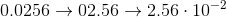### Example Question #1 : How To Convert Decimals To Scientific Notation

Convert the decimal into scientific notation: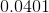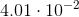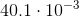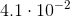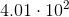Explanation:

To convert a decimal into scientific notation, move the decimal point until you get to the left of the first non-zero integer. The number of places the decimal point moves is the power of the exponent, because each movement represents a "power of 10". The exponent will be positive if the original number is greater than zero, and negative if the original number is less than zero.

For this example, move the decimal point two places to the right. Since the number is less than zero, the exponent is negative: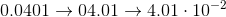### Example Question #1 : How To Convert Decimals To Scientific Notation

Convert the decimal into scientific notation: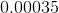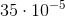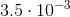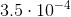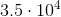Explanation:

To convert a decimal into scientific notation, move the decimal point until you get to the left of the first non-zero integer. The number of places the decimal point moves is the power of the exponent, because each movement represents a "power of 10". The exponent will be positive if the original number is greater than zero, and negative if the original number is less than zero.

For this example, move the decimal point four places to the right. Since the number is less than zero, the exponent is negative: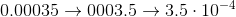### Example Question #1 : How To Convert Decimals To Scientific Notation

Convert the decimal into scientific notation: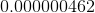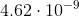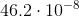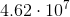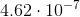Explanation:

To convert a decimal into scientific notation, move the decimal point until you get to the left of the first non-zero integer. The number of places the decimal point moves is the power of the exponent, because each movement represents a "power of 10". The exponent will be positive if the original number is greater than zero, and negative if the original number is less than zero.

For this example, move the decimal point seven places to the right. Since the number is less than zero, the exponent is negative: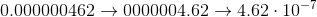### Example Question #1 : How To Convert Decimals To Scientific Notation

Convert the decimal into scientific notation: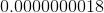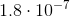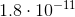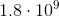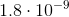Explanation:

To convert a decimal into scientific notation, move the decimal point until you get to the left of the first non-zero integer. The number of places the decimal point moves is the power of the exponent, because each movement represents a "power of 10". The exponent will be positive if the original number is greater than zero, and negative if the original number is less than zero.

For this example, move the decimal point nine places to the right. Since the number is less than zero, the exponent is negative: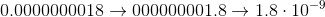### Example Question #41 : Decimals

Convert the decimal into scientific notation: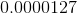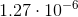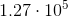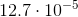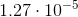Explanation:

To convert a decimal into scientific notation, move the decimal point until you get to the left of the first non-zero integer. The number of places the decimal point moves is the power of the exponent, because each movement represents a "power of 10". The exponent will be positive if the original number is greater than zero, and negative if the original number is less than zero.

For this example, move the decimal point five places to the right. Since the number is less than zero, the exponent is negative: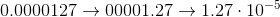← Previous 1 3

### All SAT Math Resources